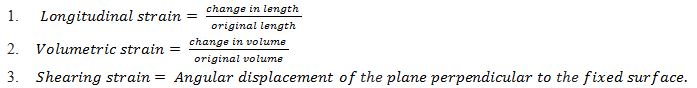The internal restoring force acting per unit area of a deformed body is called stress. If F is the force applied and A is the area of cross section of the body,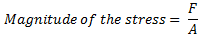The SI unit of stress is N m-2 or Pascal (Pa) and its dimensional formula is [M L-1 T-2]. Stress is a tensor quantity.

### Stress is of Two Types:

1. Normal Stress
2. Tangential Stress

There are three ways in which a solid may change its dimensions when an external force acts on it.

### Normal Stress:

In fig.1, a cylinder is stretched by two equal forces applied normal to its cross-sectional area. The restoring force per unit area, in this case, is called tensile stress. If the cylinder is compressed under the action of applied forces, the restoring force per unit area is known as compressive stress. Tensile or compressive stress can also be termed as longitudinal stress.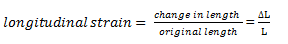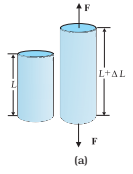Fig 1.Cylinder subjected to tensile stress stretches it by an amount ΔL.

In both the cases, there is a change in the length of the cylinder. The change in the length ∆L to the original length L of the body (cylinder in this case) is known as longitudinal strain.

### Tangential Stress:

In fig.2, if two equal and opposite deforming forces are applied parallel to the cross-sectional area of the cylinder. There is relative displacement between the opposite faces of the cylinder. The restoring force per unit area developed due to the applied tangential force is known as tangential or shearing stress and it is defined as the ratio of relative displacement of the faces Δx to the length of the cylinder L.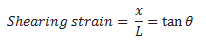where θ is the angular displacement of the cylinder from the vertical (original position of the cylinder). Usually, θ is very small, tan θ is nearly equal to angle θ, (if θ = 1 0 °, for example, there is only 1% difference between θ and tan θ).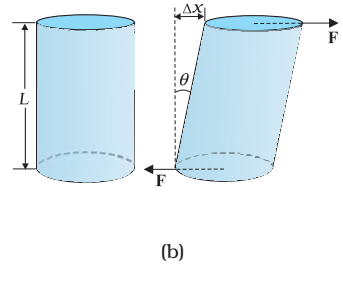Fig.2, A cylinder subjected to shearing (tangential) stress deforms by an angle θ

In Fig.3, It can also be visualized, when a book is pressed with the hand and pushed horizontally,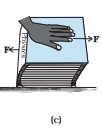Fig.3, A book subjected to a shearing stress

Thus,

In Fig.4, a solid sphere placed in the fluid under high pressure is compressed uniformly on all sides. The force applied by the fluid acts in the perpendicular direction at each point of the surface and the body is said to be under hydraulic compression. This leads to decrease in its volume without any change of its geometrical shape.

The body develops internal restoring forces that are equal and opposite to the forces applied by the fluid (the body restores its original shape and size when taken out from the fluid). The internal restoring force per unit area, in this case, is known as hydraulic stress and in magnitude is equal to the hydraulic pressure (applied force per unit area).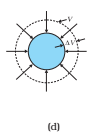Fig.4, A solid sphere subjected to a uniform hydraulic stress shrinks in volume by an amount ΔV

The strain produced by a hydraulic pressure is called volume strain and is defined as the ratio of change in volume (ΔV) to the original volume (V).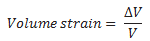Since the strain is a ratio of change in dimension to the original dimension, it has no units or dimensional formula.

In short explain Strain

The fractional change in configuration is called strain.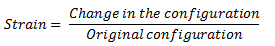It has no unit and it is a dimensionless quantity.

According to the change in configuration, the strain is of three types,